# MCQ Questions for Class 11 Chemistry Chapter 12 Organic Chemistry: Some Basic Principles and Techniques with Answers

We have compiled the NCERT MCQ Questions for Class 11 Chemistry Chapter 12 Organic Chemistry: Some Basic Principles and Techniques with Answers Pdf free download covering the entire syllabus. Practice MCQ Questions for Class 11 Chemistry with Answers on a daily basis and score well in exams. Refer to the Organic Chemistry: Some Basic Principles and Techniques Class 11 MCQs Questions with Answers here along with a detailed explanation.

## Organic Chemistry: Some Basic Principles and Techniques Class 11 MCQs Questions with Answers

Multiple Choice Type Questions

Question 1.
I.U.P.A.C. name of 4-isopropyl-m-xylene is
(a) l-Isopropyl-2, 4-Dimethyl benzene
(b) 4-Iso propyl-m-Xylene
(c) l-Isopropyl-3, 5-Dimethyl benzene
(d) 4-Isopropyl-3, 5-Dimethyl benzene.Question 2.
Among the following alkenes : 1-butane (I), cis-2-butene (II), trans-2-butene (III), the decreasing order of stability is
(a) III > II > I
(b) III > I > II.
(c) I > II > III
(d) II > I > III

Answer: (a) III > II > I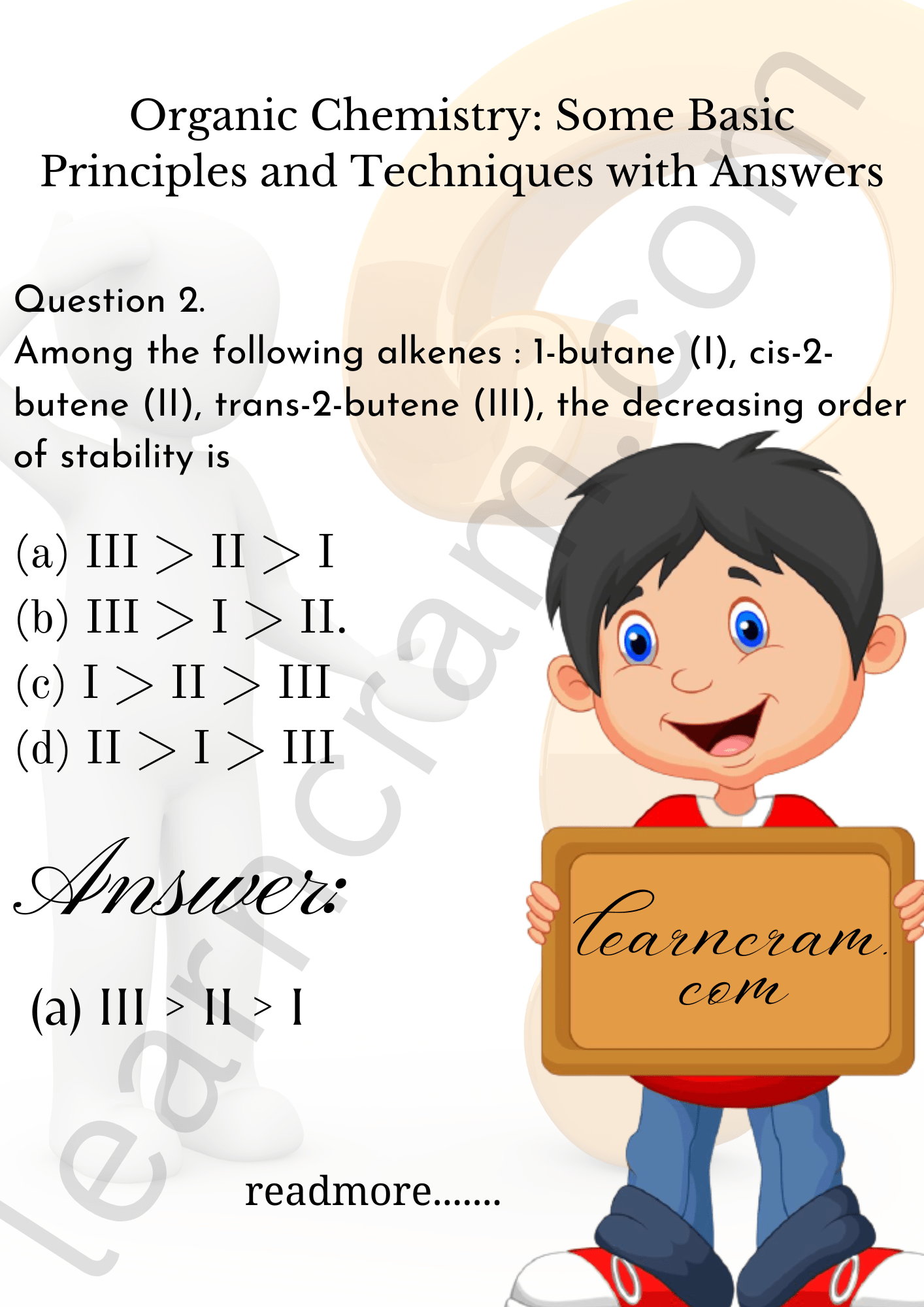Question 3.
The reaction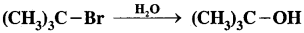is
(a) Elimination reaction
(b) Substitution reaction

Question 4.
The I.U.P.A.C. name of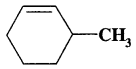(a) 3-Methyl cyclohexene
(b) 1-methyl cylohex-2-ene.
(c) 6-methyl cyclohexene
(d) 1-methyl cyclohex5-ene.

Question 5.
The I.U.P.A.C. name of CH3COCH(CH3)2 is
(a) 3-methyl-2-butanone
(b) Isopropyl methyl ketone
(c) 2-methyl-3-butanone
(d) 4-methyl isopropyl ketone

Question 6.
Which of the following compounds possess the C-H bond with the lowest bond dissociation energy?
(a) Toluene
(b) Benzene
(c) n-Pentane
(d) 2, 2-Dimethyl propane

Question 7.
The general formula CnH2nO23 could be for open chain
(a) Dialdehydes
(b) Diketones
(c) Carboxylic acid
(d) Diols

Question 8.
Name of the compound given below is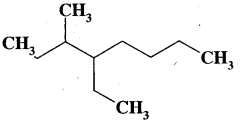(a) 4-ethyl-3-methyloctane
(b) 3 -methyl-4-ethyloctane
(c) 2, 3-diethylheptane
(d) 5 – ethyl-6 -methyloctane.

Question 9.
The C-H bond distance is longest in
(a) C2H2
(b) C2H4
(c) C2H6
(d) C2H2Br2

Question 10.
Which of the following represents the given mode of hybridisation sp²-sp²-sp-sp from left to right?
(a) CH2 = CH-C ≡ N
(b) HC ≡ C – C = CH
(c) H2C = C = C = CH2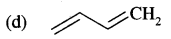Answer: (a) CH2 = CH-C ≡ N

Question 11.
What is the decreasing order of stability of the ions?
(I) CH3-CH-CH3
(II) CH3-CH-OCH3
(III) CH3-CH-CO-CH3
(a) I > II > III
(b) II > III > I
(c) III > I > II
(d) II > I > III

Answer: (d) II > I > III

Question 12.
Which of the following is an electrophile?
(a) H2O
(b) NH3
(c) AlCl3
(d) C2H5NH2

Question 13.
The most stable carbanion among the following isQuestion 14.(a) resonating structure
(b) Tautomers
(c) Geometrical isomers
(d) Optical isomers.

Question 15.
The arrangement of (CH3)3C -, (CH3)2CH-, CH3 CH2– when attached to benzene or unsaturated group in increasing order of inductive effect is
(a) (CH3)3C- < (CH3)2CH- < CH3 – CH2
(b) CH3 -CH2– < (CH3)2CH- < (CH3)3C-
(c) (CH3)2CH-< (CH3)3C- < CH3-CH2
(d) (CH3)3C- < CH3– CH2– < (CH3)2CH

Answer: (a) (CH3)3C- < (CH3)2CH- < CH3 – CH2

Question 16.
The I.U.P.A.C. name of the following compound is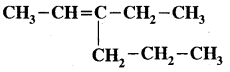(a) 3-Ethyl-2-hexene
(b) 3-Propyl-2-bexene
(c) 3-Propyl-3-hexene
(d) 4-Ethyl-4-hexene

Question 17.
The number and type of bonds between two carbon atoms in CaC2 are
(a) One sigma (σ) and one pi (π) bonds
(b) One sigma (σ) and two pi (π) bonds
(c) One sigma (σ) and one and half pi (π) bonds
(d) One sigma (σ) bond

Answer: (b) One sigma (σ) and two pi (π) bonds

Question 18.
The I.U.P.A.C name of the compond is
(a) 3, 3, 3- Trimethylpropene
(b) 1,1, 1-Trimethyl-2-pentane
(c) 3, 3-Dimethyl-l-butene
(d) 2, 2-Dimethyl-3-butane.

Question 19.
Which of the following is correct regarding the-I. Effect of substituents?
(a) -NR2 < -OR < F-
(b) -NR2 > -OR < -F
(c) -NR2 < -OR < -F
(d) -NR2 > -OR > -F

Answer: (a) -NR2 < -OR < F-

Question 20.
The I.U.P.A.C. name of CH3 – CH = CH COO C2H5 is
(a) Ethyl but-1 -enoate
(b) Ethyl but-2-enoate
(c) Ethyl prop-2-enoate
(d) None of these.

Question 21.
The I.U.P.A.C. name of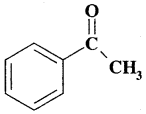(a) Acetyl cyclohexa diene
(b) l-Cyclohexa-2, 4 dienyl ethanone
(c) 6-Cyclohexa-1, 3 dienyl ethanone
(d) none of these

Answer: (b) l-Cyclohexa-2, 4 dienyl ethanone

Question 22.
CH3CH2Cl undergoes homolytic fission to produce
(a) CH3CH2 & Cl
(b) CH3CH$$_{2}^{+}$$ & Cl
(c) CH3CH$$_{2}^{+}$$ & Cl
(d) CH3CH2 & Cl

Fill in the blanks

Question 1.
A carbon atom having four different groups is known as ………………..

Question 2.
Electrophilic reagents are ……………….. & ……………….. deficient species.

Question 3.
Carbocation is formed by a group of carbon & other atoms having only ……………….. electrons.

Question 4.
Free radicals are formed due to ……………….. bond fission.

Question 5.
Mesomeric effect is observed only in the molecules having ………………..

Question 6.
Thermally unstable liquids are purified by ………………..

Question 7.
Sodium extract is boiled with HNO3 to destroy ……………….. before testing for the presence of Chlorine.

Answer: CN & / or S2-

Question 8.
In carius method, the sulphur present in the organic compound is converted into ………………..

Answer: SO$$_{4}^{2-}$$

Question 9.
The Prussian blue colour obtained during lassaigne’s test for nitrogen is due to the formation of ………………..

Question 10.
The ……………….. can be used for separating the constituents of solid mixture only when they differ in their solubilities in a solvent.

Write True / False

Question 1.
2, 2 -Dimethylbutane and 2-methylbutane are chain isomers of hexane.

Question 2.
Acetylene is a tetrahedral molecule.

Question 3.
In benzene, carbon uses all the three p orbitals for hybridisation.

Question 4.
The members of a homologous series can be represented by a general formula.

Question 5.
Propanoic acid and methyl ehtanoate are isomers.

Question 6.
Inductive effect involves displacement of electrons.

Question 7.
Electromeric effect is a permanent effect.

Question 8.
Simple carbonions are isoelectronic and isostructural with ammonia.

Question 9.
Free radicals are obtained as a result of heterolytical fission of covalent bonds.

Question 10.
Carbocations are planar species.

Match the Following